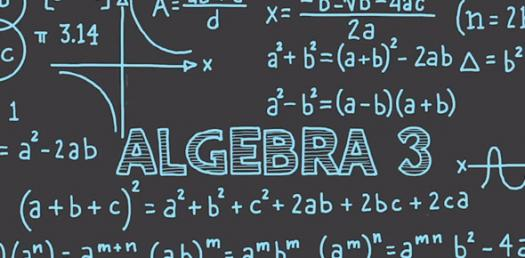# Algebra Quiz: Writing Basic Expressions Word Problems!

Approved & Edited by ProProfs Editorial Team
The editorial team at ProProfs Quizzes consists of a select group of subject experts, trivia writers, and quiz masters who have authored over 10,000 quizzes taken by more than 100 million users. This team includes our in-house seasoned quiz moderators and subject matter experts. Our editorial experts, spread across the world, are rigorously trained using our comprehensive guidelines to ensure that you receive the highest quality quizzes.
| By Cshipley
C
Cshipley
Community Contributor
Quizzes Created: 1 | Total Attempts: 1,326
Questions: 5 | Attempts: 1,329SettingsDo you know that writing basic expressions word problems will help you improve your mathematical skills? Are you unsure how to decipher expressions and equations? In mathematics, an expression is a combination of symbols that are well-formed according to the rules that rely on the context. Take this quiz to become more familiar with mathematical terms and more! Go for it!

• 1.

### Joan's cellphone company charges her \$30 a month and \$0.10 for each text she sends.  Write an expression to show Joan's cell phone bill.

• A.

\$30 = \$0.10t

• B.

\$0.10 - \$30 - t

• C.

\$30 + \$.10 + t

• D.

\$30 + \$0.10t

D. \$30 + \$0.10t
Explanation
The expression \$30 + \$0.10t represents Joan's cell phone bill. The \$30 represents the fixed monthly charge, and the \$0.10t represents the additional charge for each text she sends, where t is the number of texts. By adding these two amounts together, we get the total bill.

Rate this question:

• 2.

### Three times Cassie's age increased by nine is twelve.

• A.

3x+12 - 9

• B.

3x + 9=12

• C.

3x + 12 = 9

• D.

3x + 5

B. 3x + 9=12
Explanation
The equation 3x + 9 = 12 represents the statement "Three times Cassie's age increased by nine is twelve." This equation is formed by multiplying Cassie's age (represented by x) by three and adding nine to it, resulting in twelve.

Rate this question:

• 3.

### John sells watermelons (w) for \$4 and tangerines (t) for \$1.  Use the expression 4w + t, find the price for a customer purchasing five tangerines and two watermelons.

• A.

\$13

• B.

\$12

• C.

\$11

• D.

\$10

A. \$13
Explanation
substitute 4 in for w and 5 in for t.

Rate this question:

• 4.

### Four times the sum of nine and a number

• A.

4(9 + n)

• B.

4 x 9 + n

• C.

4 + n + 9

• D.

4n + 9

A. 4(9 + n)
Explanation
The sum means to add. You want to add the two numbers AND then multiply it by four.

Rate this question:

• 5.

### The Smith family went to the movies.  Adult tickets are \$10, kids are \$7, and senior citizens are \$5.  If the Smith family took two adults, 1 senior citizen and three kids, use an expression to show their amount spent.

• A.

\$10(4) + \$5(1) + \$7

• B.

\$10(2) + \$7(3) + \$5

• C.

\$10(2) x \$7(3) x \$5

• D.

\$10(2) + \$7(3)

B. \$10(2) + \$7(3) + \$5
Explanation
The expression \$10(2) + \$7(3) + \$5 represents the amount spent by the Smith family at the movies. The \$10(2) represents the cost of two adult tickets, the \$7(3) represents the cost of three kids' tickets, and the \$5 represents the cost of one senior citizen ticket. By multiplying the number of tickets by their respective prices and adding them together, we get the total amount spent.

Rate this question:

Related TopicsBack to top